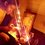# Laws of Binomial theorem (2.2)

Hello everyone , this is part 2.2 of binomial theorem ,a continuation of 2.1 where we used differentiation to get desired results . In this part we will use Integration , a complicated tool in mathematics which helps in calculating areas and averaging continuous functions .Only basic understanding of integrals and U-substitution is required to understand this note. By the way, check out wiki pages of brilliant on Definite integrals and Integration of Algebraic Functions , they are just awesome . Let's start with our cute little equation , expansion of $(1+x)^{n}$ . Let's call it equation $I$ , where $x$ is a complex number and $n$ is a whole number .

$\ (1+x)^{n} = \dbinom{n}{0}x^{0}+\dbinom{n}{1}x^{1}+\dbinom{n}{2}x^{2}+\cdots+\dbinom{n}{n-1}x^{n-1}+\dbinom{n}{n}x^{n} = \displaystyle\sum_{r=0}^{n}\dbinom{n}{r}x^{r}$

Now let's integrate it once with respect to $x$ .

$\ \int_{0}^{x}(1+x)^{n} dx = \int_{0}^{x} \dbinom{n}{0}x^{0} dx +\int_{0}^{x} \dbinom{n}{1}x^{1} dx +\cdots+\int_{0}^{x} \dbinom{n}{n}x^{n}dx = \displaystyle\sum_{r=0}^{n}\int_{0}^{x} \dbinom{n}{r}x^{r}dx$

$\dfrac{(1+x)^{n+1}-1}{n+1} = \dbinom{n}{0}\dfrac{x^{1}}{1}+\dbinom{n}{1}\dfrac{x^{2}}{2}+\cdots+\dbinom{n}{n}\dfrac{x^{n+1}}{n+1} = \displaystyle\sum_{r=0}^{n}\dbinom{n}{r}\dfrac{x^{r+1}}{r+1}$

Put $x =1$ and ....

$\boxed{ \dfrac{2^{n+1}-1}{n+1} = \dbinom{n}{0}\dfrac{1}{1}+\dbinom{n}{1}\dfrac{1}{2}+\cdots+\dbinom{n}{n}\dfrac{1}{n+1} = \displaystyle\sum_{r=0}^{n}\dbinom{n}{r}\dfrac{1}{r+1} }$

Similarly we can get loads of new series . Let's try to get

$S = \dbinom{n}{0}\dfrac{1}{1 \cdot 3}+\dbinom{n}{1}\dfrac{1}{2\cdot 4}+\cdots+\dbinom{n}{n}\dfrac{1}{(n+1)\cdot(n+3)} = \displaystyle\sum_{r=0}^{n}\dbinom{n}{r}\dfrac{1}{(r+1)\cdot(r+3)}$

Let's start with $I$

\ \begin{aligned} (1+x)^{n} & = \dbinom{n}{0}x^{0}+\dbinom{n}{1}x^{1}+\cdots+\dbinom{n}{n}x^{n} \quad \small\color{#3D99F6}{\text{0th integral}}\\ \int_{0}^{x}(1+x)^{n} dx & = \int_{0}^{x} \dbinom{n}{0}x^{0} dx +\int_{0}^{x} \dbinom{n}{1}x^{1} dx +\cdots+\int_{0}^{x} \dbinom{n}{n}x^{n}dx \\ \dfrac{(1+x)^{n+1}-1}{n+1} & = \dbinom{n}{0}\dfrac{x^{1}}{1}+\dbinom{n}{1}\dfrac{x^{2}}{2}+\cdots+\dbinom{n}{n}\dfrac{x^{n+1}}{n+1} \quad \small\color{#3D99F6}{\text{1st integral}} \\ \dfrac{x(1+x)^{n+1}-x}{n+1} & = \dbinom{n}{0}\dfrac{x^{2}}{1}+\dbinom{n}{1}\dfrac{x^{3}}{2}+\cdots+\dbinom{n}{n}\dfrac{x^{n+2}}{n+1} \quad \small\color{#3D99F6}{\text{times x}} \\ \int_{0}^{x} \dfrac{x(1+x)^{n+1}-x}{n+1}dx & = \int_{0}^{x} \dbinom{n}{0}\dfrac{x^{2}}{1}dx +\int_{0}^{x}\dbinom{n}{1}\dfrac{x^{3}}{2}dx+\cdots+\int_{0}^{x}\dbinom{n}{n}\dfrac{x^{n+2}}{n+1}dx \\ \dfrac{1}{n+1}\int_{0}^{x}x(1+x)^{n+1}dx -\dfrac{x^2}{2(n+1)}& = \dbinom{n}{0}\dfrac{x^3}{1 \cdot 3}+\dbinom{n}{1}\dfrac{x^4}{2\cdot 4}+\cdots+\dbinom{n}{n}\dfrac{x^{n+1}}{(n+1)\cdot(n+3)} \quad \small\color{#3D99F6}{\text{2nd integral}} \\ \dfrac{1}{n+1}\underbrace{\int_{0}^{1}x(1+x)^{n+1}dx}_{I_{1}} -\dfrac{1}{2(n+1)} & = \dbinom{n}{0}\dfrac{1}{1 \cdot 3}+\dbinom{n}{1}\dfrac{1}{2\cdot 4}+\cdots+\dbinom{n}{n}\dfrac{1}{(n+1)\cdot(n+3)} \quad \small\color{#3D99F6}{\text{put x=1}} \end{aligned}

\ \begin{aligned} I_{x} & = \int_{0}^{x}x(1+x)^{n+1}dx \quad \quad \small\text{put } 1+x=t\\ & = \int_{1}^{x+1} (t-1)t^{n+1}dt \\ & = \int_{1}^{x+1} t^{n+2}-t^{n+1}dt \\ I_{x} & = \dfrac{(x+1)^{n+3}}{n+3}-\dfrac{(x+1)^{n+2}}{n+2}-\dfrac{1}{n+3}+\dfrac{1}{n+2}\\ I_{1} & = \dfrac{2^{n+3}-1}{n+3}-\dfrac{2^{n+2}-1}{n+2} \end{aligned}

$\ \dfrac{1}{n+1}\left( \dfrac{2^{n+3}-1}{n+3}-\dfrac{2^{n+2}-1}{n+2} \right) -\dfrac{1}{2(n+1)} = \dbinom{n}{0}\dfrac{1}{1 \cdot 3}+\dbinom{n}{1}\dfrac{1}{2\cdot 4}+\cdots+\dbinom{n}{n}\dfrac{1}{(n+1)\cdot(n+3)}$

$\ \boxed{ \dfrac{2^{n+3}(2n+1)-n^2-5n-4}{2(n+1)(n+2)(n+3)} = \dbinom{n}{0}\dfrac{1}{1 \cdot 3}+\dbinom{n}{1}\dfrac{1}{2\cdot 4}+\cdots+\dbinom{n}{n}\dfrac{1}{(n+1)\cdot(n+3)} }$

For Practice try to find the sum of the following series:

$1) \hspace{2mm} \dbinom{n}{0}\dfrac{1}{1\cdot 2}+\dbinom{n}{1}\dfrac{1}{2\cdot 3}+\cdots+\dbinom{n}{n}\dfrac{1}{(n+1)\cdot(n+2)} = \displaystyle\sum_{r=0}^{n}\dbinom{n}{r}\dfrac{1}{(r+1)(r+2)}$

$2) \hspace{2mm} \dbinom{n}{0}\dfrac{1}{1^2}+\dbinom{n}{1}\dfrac{1}{2^2}+\cdots+\dbinom{n}{n}\dfrac{1}{(n+1)^2} = \displaystyle\sum_{r=0}^{n}\dbinom{n}{r}\dfrac{1}{(r+1)^2}$

I Hope you enjoyed this note and If someone knows the answer to 2nd problem please post it in the comments section below. thank you :) . And Merry Christmas everyone !!!Note by Sabhrant Sachan
3 years, 10 months ago

This discussion board is a place to discuss our Daily Challenges and the math and science related to those challenges. Explanations are more than just a solution — they should explain the steps and thinking strategies that you used to obtain the solution. Comments should further the discussion of math and science.

When posting on Brilliant:

• Use the emojis to react to an explanation, whether you're congratulating a job well done , or just really confused .
• Ask specific questions about the challenge or the steps in somebody's explanation. Well-posed questions can add a lot to the discussion, but posting "I don't understand!" doesn't help anyone.
• Try to contribute something new to the discussion, whether it is an extension, generalization or other idea related to the challenge.

MarkdownAppears as
*italics* or _italics_ italics
**bold** or __bold__ bold
- bulleted- list
• bulleted
• list
1. numbered2. list
1. numbered
2. list
Note: you must add a full line of space before and after lists for them to show up correctly
paragraph 1paragraph 2

paragraph 1

paragraph 2

[example link](https://brilliant.org)example link
> This is a quote
This is a quote
    # I indented these lines
# 4 spaces, and now they show
# up as a code block.

print "hello world"
# I indented these lines
# 4 spaces, and now they show
# up as a code block.

print "hello world"
MathAppears as
Remember to wrap math in $$ ... $$ or $ ... $ to ensure proper formatting.
2 \times 3 $2 \times 3$
2^{34} $2^{34}$
a_{i-1} $a_{i-1}$
\frac{2}{3} $\frac{2}{3}$
\sqrt{2} $\sqrt{2}$
\sum_{i=1}^3 $\sum_{i=1}^3$
\sin \theta $\sin \theta$
\boxed{123} $\boxed{123}$

Sort by:

The problem burns down to $\displaystyle \int_{0}^{1} \dfrac{(1+x)^{n+1} -1}{x(n+1)} dx$

- 3 years, 10 months ago

Exactly I went a little ahead and got this to be s=1/(n+1)*(1+sum (2^r-1^r)/r)where r varies from 2 to n+1.

- 3 years, 10 months ago